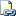# TIMEFROMPARTS (Transact-SQL)

Returns a time value for the specified time and with the specified precision.Transact-SQL Syntax Conventions

## Syntax

``````TIMEFROMPARTS ( hour, minute, seconds, fractions, precision )
``````

## Arguments

• hour
Integer expression specifying hours.

• minute
Integer expression specifying minutes.

• seconds
Integer expression specifying seconds.

• fractions
Integer expression specifying fractions.

• precision
Integer literal specifying the precision of the time value to be returned.

## Return Types

time ( precision )

## Remarks

TIMEROMPARTS returns a fully initialized time value. If the arguments are invalid, then an error is raised. If any of the parameters are null, null is returned. However, if the precision argument is null, then an error is raised.

The fractions argument depends on the precision argument. For example, if precision is 7, then each fraction represents 100 nanoseconds; if precision is 3, then each fraction represents a millisecond. If the value of precision is zero, then the value of fractions must also be zero; otherwise, an error is raised.

This function can be remoted to SQL Server 2012 servers and higher. It cannot be remoted to servers that have a version lower thanSQL Server 2012.

## Examples

### A. Simple example without fractions of a second

``````SELECT TIMEFROMPARTS ( 23, 59, 59, 0, 0 ) AS Result;
``````

Here is the result set.

``````Result
--------------------
23:59:59.0000000

(1 row(s) affected)
``````

### B. Example with fractions of a second

The following example demonstrates the use of the fractions and precision parameters:

1. When fractions has a value of 5 and precision has a value of 1, then the value of fractions represents 5/10 of a second.

2. When fractions has a value of 50 and precision has a value of 2, then the value of fractions represents 50/100 of a second.

3. When fractions has a value of 500 and precision has a value of 3, then the value of fractions represents 500/1000 of a second.

``````SELECT TIMEFROMPARTS ( 14, 23, 44, 5, 1 );
SELECT TIMEFROMPARTS ( 14, 23, 44, 50, 2 );
SELECT TIMEFROMPARTS ( 14, 23, 44, 500, 3 );
GO
``````

Here is the result set.

``````----------------
14:23:44.5

(1 row(s) affected)

----------------
14:23:44.50

(1 row(s) affected)

----------------
14:23:44.500

(1 row(s) affected)
``````